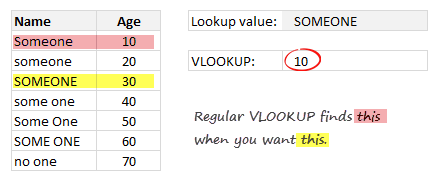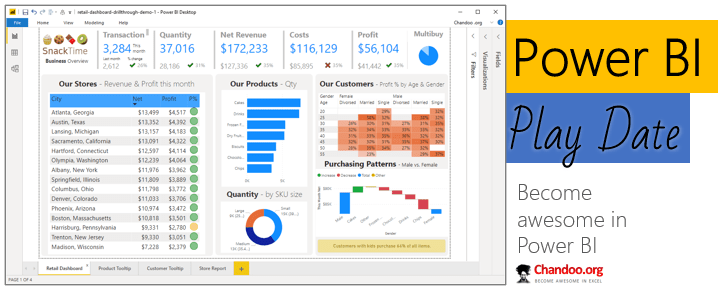# Case Sensitive Lookups

Share

We all know that VLOOKUP (and its cousins MATCH, HLOOKUP and LOOKUP) are great for finding information you want. But they are helpless when you want to do a case-sensitive lookup.So how do we write case sensitive VLOOKUP formulas?

Simple. We can use EXACT formula.

### What exactly is the EXACT formula?

EXACT formula checks if 2 cells have exactly the same value. And it is very SenSITive.

For example, `=EXACT("this","THIS")` will be false , where as =”this”=”THIS” will be true.

### Using EXACT formula to do case sensitive lookups

Let’s say the value you are looking up is in cell F4, the lookup range is B5:C11 (column B has lookup value and column C has value you want).

You can use EXACT formula along with INDEX + MATCH or SUMPRODUCT to do case sensitive lookup. Let’s look at each of these variations:

Using EXACT & INDEX + MATCH formulas to do case sensitive lookups:

Formula: `{=INDEX(\$C\$5:\$C\$11,MATCH(TRUE,EXACT(\$F\$4,\$B\$5:\$B\$11),0))}`

How it works?

Let’s go from inside out.

`EXACT(F4, B5:B11)` portion: This will return an array of TRUE & FALSE values. Something like this:

```{FALSE;FALSE;TRUE;FALSE;FALSE;FALSE;FALSE} ```
`MATCH(TRUE, EXACT(...), 0)` portion: Now we look for TRUE in all the values EXACT has returned. This will be 3 (since 3rd value in the array is true).

`INDEX(C5:C11, MATCH(...))` portion:  This will simply return the 3rd value in the column C, ie an exact match.

`{INDEX(...)}`:  Because this is an array formula, you must press CTRL+Shift+Enter after typing it. The {} indicates this.

Using EXACT + SUMPRODUCT formula:

If the lookup result is a number (or date) and there is only matching value, you can use SUMPRODUCT to do case sensitive lookups.

Formula:`=SUMPRODUCT(EXACT(\$F\$4,\$B\$5:\$B\$11) * (\$C\$5:\$C\$11))`

How it works?

The `EXACT(F4, B5:B11)` portion returns a bunch of TRUE & FALSE values.

When you multiply these TRUE & FALSE values with column C (which contains numbers), the end result will be the value you are looking for.

This is possible because in Excel, TRUE is 1 and FALSE is 0. So when you multiply a list of logical values (true / false) with a list of numbers, everything that corresponds to false becomes 0.

So we get,

`{0;0;30;0;0;0;0}`

SUMPRODUCT simply adds up these numbers and returns 30 as result.

Note: This formula won’t work if you have text values in column C or more than one TRUE in EXACT result (ie multiple values match the lookup criteria).

### More ways to lookup:

Get The VLOOKUP Book: If you are always looking for help about VLOOKUP, look no further. Get my book, it’s going to make you awesome in VLOOKUP, INDEX+MATCH, multi-condition lookups, 2 way lookups and more. Click here to order your copy.

### How do you write case sensitive lookups?

Let me be honest. I haven’t had a single case sensitive lookup scenario in last year. But email from a reader prompted me to research this problem.

What about you? Do you often deal with case-sensitive data? How do you write case sensitive lookups? Please share your tips & formulas in comments section.

### Get FREE Excel + Power BI Tips

Simple, fun and useful emails, once per week.

Learn & be awesome.

### Welcome to Chandoo.org

Thank you so much for visiting. My aim is to make you awesome in Excel & Power BI. I do this by sharing videos, tips, examples and downloads on this website. There are more than 1,000 pages with all things Excel, Power BI, Dashboards & VBA here. Go ahead and spend few minutes to be AWESOME.

Read my storyFREE Excel tips book

Chandoo is an awesome teacher
5/5

– Jason

Still on fence about Power BI? In this getting started guide, learn what is Power BI, how to get it and how to create your first report from scratch.### Introducing Power BI Play Date – Online class to master Power BI (oh yeah, Power Query & Power Pivot too)

Hello friends,

Check out below slideshow to see some of the reports you can create in our course. It is #AWESOME.

## Related Tips

### 3 Responses to “Case Sensitive Lookups”

1.jason m says:

Alternative:

=LOOKUP(2,1/EXACT(F4,B5:B11),C5:C11)

2.Gijs says:

I have a related question, hopefully someone can figure this out:

Is it possible to do a MATCH on TWO columns?
I want to copy the SalesRep name for an account in a specific country (eg IBM Germany). So i would have to do an INDEX with a MATCH on both ACCTNAME and ACCTCOUNTRY.

Can that be done?
Thanx!

3.Asheesh says:

@Gijs

You should ideally post your question in the forum...however, one of the possible solutions for your question is

INDEX(SalesRep,MATCH(1,(Country_Range="Germany")*(Account_Range="IBM"),0))

To be entered with CTRL + SHIFT +ENTER

There are several methods explained in the below link

http://dailydoseofexcel.com/archives/2009/04/21/vlookup-on-two-columns/

### Get FREE Excel & Power-BI Newsletter

One email per week with Excel and Power BI goodness. Join 100,000+ others and get it free.